Submit a blog to KDnuggets -- Top Blogs Win A Reward

Topics: AI | Data Science | Data Visualization | Deep Learning | Machine Learning | NLP | Python | R | Statistics

KDnuggets Home » News » 2017 » Jul » Tutorials, Overviews » CAN (Creative Adversarial Network) - Explained ( 17:n27 )

# CAN (Creative Adversarial Network) - Explained

http likes 78

GANs (Generative Adversarial Networks), a type of Deep Learning networks, have been very successful in creating non-procedural content. This work explores the possibility of machine generated creative content.

Lately, GANs (Generative Adversarial Networks) have been really successful in creating interesting content that are fairly abstract and hard to create procedurally. This paper, aptly named CAN (Creative, instead of Generative, Adversarial Networks) explores the possibility of machine generated creative content.

The original paper can be found here

This article assumes familiarity with neural networks, and essential aspects of them, including Loss Functions and Convolutions.### How this article is structured

I will follow the paper’s structure as much as I can. I will add my own bits to help better understand the material.

### GAN Recap

GAN’s consists of two competing neural networks, namely the Generator and the Discriminator. As is suggestive of the name, the Generator is responsible for generating data from some input (this input can be noise or even some other data). The discriminator is then responsible for analysing that data and discriminating wether that data was real(if it came from our dataset) or if its fake(if it came from the generator). Formally, it can be seen as a minimax game played by the Generator and the Discriminator like so: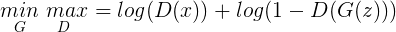### Relax:

If the above equation was too complex for you, you are not alone. I will go through this equation step by step and explain what each component means.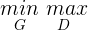This is the notation for the MiniMax Equation. The G and D subscripts stand for Generator and Discriminator respectively. It is the Generator’s job to Minimise the value of Equation 1.0 , while it is the Discriminator’s job to Maximise it. They both compete with each other endlessly(till we decide to stop).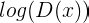The output of Discriminator for data it thought were real , when given input x (x is data from our real dataset).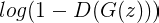This calculates how the discriminator did on the input from the generator. D(G(z)) denotes the data that the discriminator thought were real. Doing 1- D(G(z)) denotes the data the discriminator thought were NOT real. G(z) Denotes the data generated by the generator.

Putting it all together , its the Discriminator’s job to make value of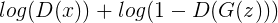As large as possible, while it is the Generator’s job to make the value of Equation 1.4 as small as possible by Maximizing the value of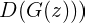A more detailed explanation can be found at http://wiki.ubc.ca/Course:CPSC522/Generative_Adversarial_Networks

Intuitive definition
The Generator will try to modify itself to make the Discriminator pass its own creation as real, while the Discriminator will modify itself to be able to continue telling the difference.

### But isn’t this just plain imitation ?

Yes it is! Note that it is the generator’s objective to fool the Discriminator into thinking that the data it generated matches the real data as much as possible. So whats the best way to do it? To make it’s outputs look very much like the real data!

This is a problem if you want your network to be creative. Your generator will not learn to create new content, but it will just try to make its output look like the real data.

### Solution? Creative Adversarial Networks

The authors propose a modified GAN to generate creative content. They propose sending an additional signal to the generator to prevent it from generating content that is too similar to existing content. How did they do it? They modified the original GAN loss function from Equation 1.4.

### Intuitive explain of CAN

In the original GAN, the generator modifies its weights based on the discriminator’s output of wether or not what it generated was able to fool the discriminator. CAN extends this in two ways:

1. The discriminator will not only discriminate if it thinks the data is real or fake, but additionally will also classify which time period the artwork belongs to.
2. The Generator will take in the additional information about the time period from the discriminator , and use that metric along with real/fake input from the Discriminator.

### What is the point of doing this?

The original problem of GAN was they would not explore new work. Their objective is literally to just make their data look like it came from real dataset.

By having an additional metric which classifies the time period the data belongs to(along with the confidence), the generator is now getting feedback on how similar it’s creation looks to some time period.

Now, the generator not only has to make its data look similar to dataset, but also make sure it doesn’t look too similar to a single category. This will allow it to prevent creating artwork that has very specific characteristics.
The new loss function is:Its really simple!

The first line is exactly the same as the original equation. Note that the subscript r means the discriminator’s output of real/fake, and the subscript c is the output of the discriminator’s classification.
The 2nd line is the modification for promoting creativity. I will explain it step by step.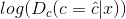This is the Discriminator getting the class of the input image correctly. The Discriminator will try to maximize this value. We want the discriminator to classify the images correctly.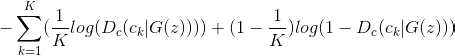This may look complicated , but this is just the Multi Label Cross Entropy Loss. Note that K here denotes the number of classes. You can find the detailed information about losses here. This is the same loss that classifiers use as a loss function. The generator will try to minimize this value in order to maximise Equation 2.0.

### Intuitive explanation of Equation 2.2

The way that Equation 2.2 works is , if the value of one of the classes score approaches 1 or 0 , the value of the whole equation approaches -infinity. The largest possible value(larger is what the generator wants) that Equation 2.2 can take is when the discriminator is completely unsure about what class the input belongs to, i.e. every term in the summation has the same value. This makes sense because its not possible to properly classify the input image into existing classes, so it must mean that it is its own new class.

### Conclusion

This paper talks about a loss function that pushes a GAN into exploring new content based on what is given to it. This was done by modifying the loss function to allow for exploration.

### P.S.

This was my first technical post. Criticism and improvement tips are welcome and greatly appreciated.
If you learned something useful from my article, please share it with others. It lets me know I was of help.

Original. Reposted with permission.

Bio: Harshvardhan Gupta writes at HackerNoon.

Related:

## Top Stories Past 30 Days

Most PopularGet KDnuggets, a leading newsletter on AI, Data Science, and Machine Learning

By subscribing you accept KDnuggets Privacy Policy